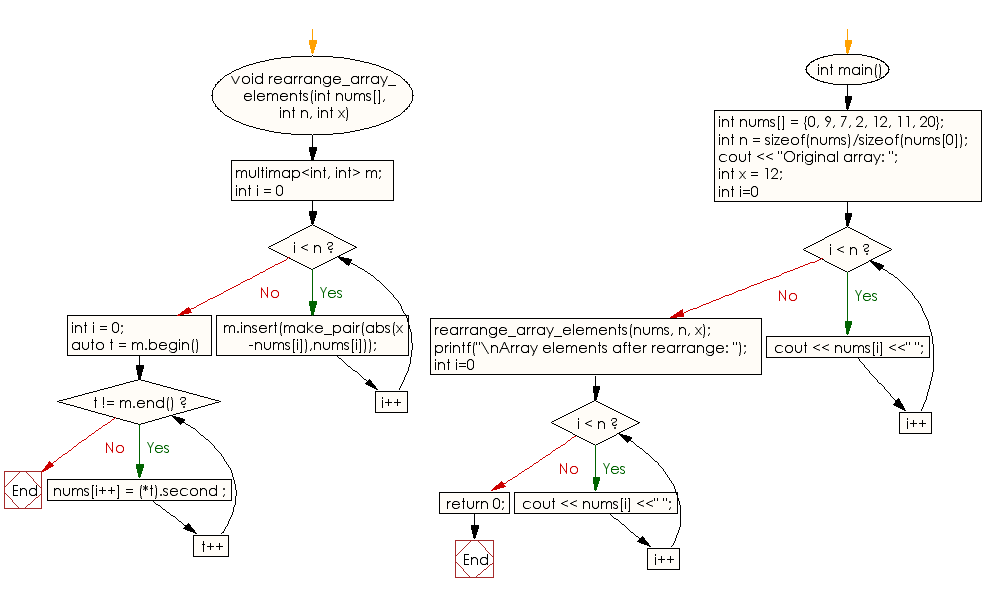﻿ C++ : Sort an array of distinct values according to a value# C++ Exercises: Sort an array of distinct elements according to absolute difference of array elements and with a given value

## C++ Array: Exercise-17 with Solution

Write a C++ program to sort (in descending order) an array of distinct elements according to absolute difference of array elements and with a given value.

Sample Solution:

C++ Code :

``````#include <iostream>
using namespace std;

void rearrange_array_elements(int nums[], int n, int x)
{
multimap<int, int> m;

for (int i = 0 ; i < n; i++)
m.insert(make_pair(abs(x-nums[i]),nums[i]));

int i = 0;
for (auto t = m.begin(); t != m.end(); t++)
nums[i++] = (*t).second ;
}

int main()
{
int nums[] = {0, 9, 7, 2, 12, 11, 20};
int n = sizeof(nums)/sizeof(nums);
cout << "Original array: ";
int x = 12;
for (int i=0; i < n; i++)
cout << nums[i] <<" ";
rearrange_array_elements(nums, n, x);

printf("\nArray elements after rearrange: ");
for (int i=0; i < n; i++)
cout << nums[i] <<" ";
return 0;

}
``````

Sample Output:

```Original array: 0 9 7 2 12 11 20
Array elements after rearrange: 12 11 9 7 20 2 0
```

Flowchart:C++ Code Editor:

What is the difficulty level of this exercise?

Test your Programming skills with w3resource's quiz.

﻿